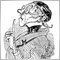CopyRates function returning -1 with error 4401 : Different behavior between two launched.15

Good morning,

I had a strange behavior using the CopyRates function in MQL5. I tried to load some Rates using the CopyRates function for a specified Symbol (CustomSymbol). The requested data is on a PERIOD_M1 and represents two months of rates starting from the SERIES_TERMINAL_FIRSTDATE of the Symbol.

The first time I ran the script, the function always returns -1 with the error code 4401 (from GetLastError()). The second time I ran the script with the same set of parameters, it worked perfectly. It seems that MT5 loaded the data asynchronously between the two launches.

The source code:

bool bCheckSymbolRates(string sSymbol, datetime oStartDateTime, datetime oEndDateTime)
{
bool  bRet = true;
int   _iSourceMqlRatesCount = 0;

// Checks minimum first date
if (oStartDateTime < (datetime) SeriesInfoInteger(sSymbol, 0, SERIES_TERMINAL_FIRSTDATE))
oStartDateTime = (datetime) SeriesInfoInteger(sSymbol, 0, SERIES_TERMINAL_FIRSTDATE);

// dynamic table allocation
_iSourceMqlRatesCount = 0;

while (bRet == true && oStartDateTime < oEndDateTime)
{
// 2 months of data
datetime  oTmpEndDateTime = oStartDateTime + 3600 * 24 * 61;
MqlRates  tTmpMqlRates[];

ArraySetAsSeries(tTmpMqlRates, false);
int  iCount = CopyRates(sSymbol, PERIOD_M1, oStartDateTime, oTmpEndDateTime, tTmpMqlRates);

if (iCount != -1)
{
datetime oFirstvalue = tTmpMqlRates.time;
_iSourceMqlRatesCount += iCount;
}
else
{
int iError = GetLastError();
printf("AggSymbolData - Error while loading data : %i", GetLastError());
bRet = false;
}

// Go to the next range
oStartDateTime = oTmpEndDateTime + 60;
}
return bRet;
};

1st launch: FAILED

SERIES_TERMINAL_FIRSTDATE = D'2003.03.24 00:00:00'

SERIES_FIRSTDATE = D'2003.03.24 09:15:00'

SERIES_LASTBAR_DATE = D'2018.02.08 21:45:00'

SERIES_BARS_COUNTS = 203018

SERIES_SYNCHRONIZED = TRUE

iCount = -1 and iError = 4401

2nd launch:  SUCCESS with the same set of parameters

SERIES_TERMINAL_FIRSTDATE = D'2003.03.24 00:00:00'

SERIES_FIRSTDATE = D'2003.03.24 09:15:00'

SERIES_LASTBAR_DATE = D'2018.02.08 21:45:00'

SERIES_BARS_COUNTS = 203018

SERIES_SYNCHRONIZED = TRUE

iCount = 27427 (on the first while iteration).

Is there a solution to avoid this problem like?

ThanksModerator
8499

Just look into the reference!

You'll find: ERR_HISTORY_NOT_FOUND which means that your terminal hasn't got all the quotes it has requested.-

Either: if (..) return() or if(..) sleep().39020

fdelaitre:

Good morning,

I had a strange behavior using the CopyRates function in MQL5. I tried to load some Rates using the CopyRates function for a specified Symbol (CustomSymbol). The requested data is on a PERIOD_M1 and represents two months of rates starting from the SERIES_TERMINAL_FIRSTDATE of the Symbol.

The first time I ran the script, the function always returns -1 with the error code 4401 (from GetLastError()). The second time I ran the script with the same set of parameters, it worked perfectly. It seems that MT5 loaded the data asynchronously between the two launches.

The source code:

1st launch: FAILED

SERIES_TERMINAL_FIRSTDATE = D'2003.03.24 00:00:00'

SERIES_FIRSTDATE = D'2003.03.24 09:15:00'

SERIES_LASTBAR_DATE = D'2018.02.08 21:45:00'

SERIES_BARS_COUNTS = 203018

SERIES_SYNCHRONIZED = TRUE

iCount = -1 and iError = 4401

2nd launch:  SUCCESS with the same set of parameters

SERIES_TERMINAL_FIRSTDATE = D'2003.03.24 00:00:00'

SERIES_FIRSTDATE = D'2003.03.24 09:15:00'

SERIES_LASTBAR_DATE = D'2018.02.08 21:45:00'

SERIES_BARS_COUNTS = 203018

SERIES_SYNCHRONIZED = TRUE

iCount = 27427 (on the first while iteration).

Is there a solution to avoid this problem like?

Thanks

There is no problem at all, it's how it works.19837

On MT5: Unless the chart is that specific pair/TF, you must Synchronize the terminal Data from the Server.
Timeseries and Indicators Access /  Data Access - Reference on algorithmic/automated trading language for MetaTrader 5